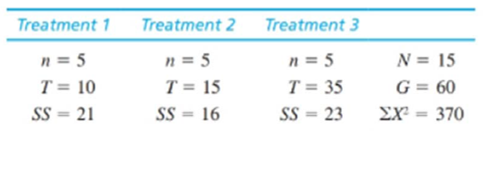Chapter 12.3, Problem 1LCEssentials of Statistics for the B...

8th Edition
Frederick J Gravetter + 1 other
ISBN: 9781133956570

Solutions

Chapter
SectionEssentials of Statistics for the B...

8th Edition
Frederick J Gravetter + 1 other
ISBN: 9781133956570
Textbook Problem

Calculate SStotal, SSbetween, and SSwithin for the following set of data:To determine

To calculate: The values of SStotal,SSbetween and SSwithin for the dataset provided.

Explanation

Given info:

The dataset with sample size (n), treatment total (T), sum of squared difference (SS), sum of scores (X=G),X2 and N is provided for three treatments.

Calculations:

Total sum of squares:

SStotal=X2(X)2N=X2G2N

Substituting the values in the above equation

SStotal=37060215SStotal=130

Between sum of squares:

SSbetween=T2nG2N

Substituting the respective values in the equation

SSbetween=1025+15

Still sussing out bartleby?

Check out a sample textbook solution.

See a sample solution

The Solution to Your Study Problems

Bartleby provides explanations to thousands of textbook problems written by our experts, many with advanced degrees!

Get Started

Define the terms population and sample, and explain the role of each in a research study.

Essentials of Statistics for The Behavioral Sciences (MindTap Course List)

Sketch the region in the xy-plane. 50. {(x, y) | y 2x 1}

Single Variable Calculus: Early Transcendentals, Volume I

Find the limit. limx0cscxsin(sinx)

Single Variable Calculus: Early Transcendentals

Domain Find the domain of the function. 20. k(x) = ln | x |

Precalculus: Mathematics for Calculus (Standalone Book)

The polar equation of the conic with eccentricity 3 and directrix x = −7 is:

Study Guide for Stewart's Single Variable Calculus: Early Transcendentals, 8th

The vector represented by where A(4, 8) and B(6, 6)is:

Study Guide for Stewart's Multivariable Calculus, 8th